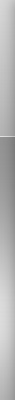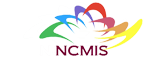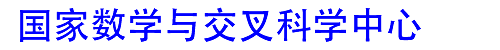网站地图 | 联系我们 | English | 意见反馈 | 主任信箱
 首页中心概况新闻动态科研进展交流合作人才培养研究队伍人才招聘政策规章数学交叉科学传播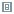科研进展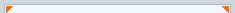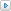科研成果研究专题获奖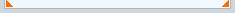现在位置：首页 > 科研进展 > 科研成果
 气态星云的连续引力塌缩及零压欧拉-泊松方程 【大 中 小】【打印】【关闭】 2022-9-12The gravitational collapse of an isolated self-gravitating gaseous star for $\gamma$-law pressure $p(\rho)=\rho^\gamma$ ($1<\gamma<\frac43$) in the mass-supcritical case is investigated. It was first shown in [Y. Guo, M. Had？i？, and J. Jang, Arch. Rational Mech. Anal., 239 (2021), pp. 431--552] that there exists a kind of continued gravitational collapse, and the collapse is based on a special solution of the pressureless Euler--Poisson system. In this paper, all spherically symmetric solutions of the pressureless Euler--Poisson system are classified. Precisely speaking, for fixed radius $r$, there exists a unique critical velocity $v^*(r)>0$ depending on the mean density in the ball $B(0,r)$ for the pressureless Euler--Poisson system such that if the initial velocity $\chi_1(r)\geq v^*(r)$ (escape case), then the dust runs away from the gravitational force forever along an escape trajectory, and if the initial velocity $\chi_1(r)< v^*(r)$ (collapse case), then the dust collapses at the origin in a finite time $t^*(r)$ even if it expands initially, i.e., $\chi_1(r)>0$. Moreover, it is proved that there exists a class of spherically symmetric solutions of a gaseous star, which formulates a continued gravitational collapse in finite time, based on the background of the pressureless solutions if $\chi_1(r)< v^*(r)$ for all $r\in[0,1]$. It is noted that $\chi_1(r)$ could be positive; that is, the star might expand initially but finally collapse.　　　　 Publication:  SIAM Journal on Mathematical Analysis. Volume. 54, Issue. 3, pp: 3139-3160.  Author:   Feimin HuangAcademy of Mathematics and System Sciences, Chinese Academy of Sciences, Beijing 100190, China, and School of Mathematical Sciences, University of Chinese Academy of Sciences, Beijing 100049, ChinaEmail: fhuang@amt.ac.cn Yue YaoAcademy of Mathematics and System Sciences, Chinese Academy of Sciences, Beijing 100190, China, and School of Mathematical Sciences, University of Chinese Academy of Sciences, Beijing 100049, ChinaEmail: yaoyue17@mails.ucas.ac.cn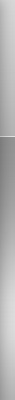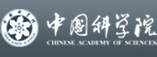欢迎访问国家数学与交叉科学中心　 地址：北京海淀区中关村东路55号　邮编：100190　电话: 86-10-62613242 Fax: 86-10-62616840 邮箱: ncmis@amss.ac.cn